# Recent Activity

## Monochromatic reachability or rainbow triangles ★★★

Author(s): Sands; Sauer; Woodrow

In an edge-colored digraph, we say that a subgraph is rainbow if all its edges have distinct colors, and monochromatic if all its edges have the same color.

Problem   Letbe a tournament with edges colored from a set of three colors. Is it true thatmust have either a rainbow directed cycle of length three or a vertexso that every other vertex can be reached fromby a monochromatic (directed) path?

Keywords: digraph; edge-coloring; tournament

## Rank vs. Genus ★★★

Author(s): Johnson

Question   Is there a hyperbolic 3-manifold whose fundamental group rank is strictly less than its Heegaard genus? How much can the two differ by?

Keywords:

## The Hodge Conjecture ★★★★

Author(s): Hodge

Conjecture   Letbe a complex projective variety. Then every Hodge class is a rational linear combination of the cohomology classes of complex subvarieties of.

Keywords: Hodge Theory; Millenium Problems

## 2-accessibility of primes ★★

Author(s): Landman; Robertson

Question   Is the set of prime numbers 2-accessible?

Keywords: monochromatic diffsequences; primes

## Non-edges vs. feedback edge sets in digraphs ★★★

Author(s): Chudnovsky; Seymour; Sullivan

For any simple digraph, we letbe the number of unordered pairs of nonadjacent vertices (i.e. the number of non-edges), andbe the size of the smallest feedback edge set.

Conjecture  Ifis a simple digraph without directed cycles of length, then.

Keywords: acyclic; digraph; feedback edge set; triangle free

## Tarski's exponential function problem ★★

Author(s): Tarski

Conjecture   Is the theory of the real numbers with the exponential function decidable?

Keywords: Decidability

## Jacobian Conjecture ★★★

Author(s): Keller

Conjecture   Letbe a field of characteristic zero. A collectionof polynomials in variablesdefines an automorphism of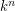if and only if the Jacobian matrix is a nonzero constant.

Keywords: Affine Geometry; Automorphisms; Polynomials

## Counting 3-colorings of the hex lattice ★★

Author(s): Thomassen

Problem   Find.

Keywords: coloring; Lieb's Ice Constant; tiling; torus

## Dense rational distance sets in the plane ★★★

Author(s): Ulam

Problem   Does there exist a dense set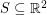so that all pairwise distances between points inare rational?

Keywords: integral distance; rational distance

## Negative association in uniform forests ★★

Author(s): Pemantle

Conjecture   Letbe a finite graph, let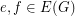, and let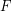be the edge set of a forest chosen uniformly at random from all forests of. Then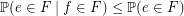Keywords: forest; negative association

## Complete bipartite subgraphs of perfect graphs ★★

Author(s): Fox

Problem   Letbe a perfect graph onvertices. Is it true that eitheror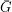contains a complete bipartite subgraph with bipartition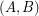so that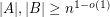?

Keywords: perfect graph

## Wall-Sun-Sun primes and Fibonacci divisibility ★★

Author(s):

Conjecture   For any prime, there exists a Fibonacci number divisible byexactly once.

Equivalently:

Conjecture   For any prime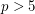,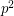does not dividewhere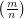is the Legendre symbol.

Keywords: Fibonacci; prime

## A discrete iteration related to Pierce expansions ★★

Author(s): Shallit

Conjecture   Let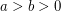be integers. Set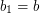andfor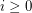. Eventually we have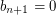; put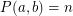.

Example: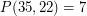, since,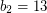,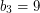,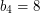,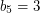,,,.

Prove or disprove: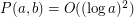.

Keywords: Pierce expansions

## Total Colouring Conjecture ★★★

Conjecture   A total coloring of a graphis an assignment of colors to the vertices and the edges ofsuch that every pair of adjacent vertices, every pair of adjacent edges and every vertex and incident edge pair, receive different colors. The total chromatic number of a graph,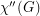, equals the minimum number of colors needed in a total coloring of. It is an old conjecture of Behzad that for every graph, the total chromatic number equals the maximum degree of a vertex in,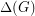plus one or two. In other words,Keywords: Total coloring

## Edge Reconstruction Conjecture ★★★

Author(s): Harary

Conjecture

Every simple graph with at least 4 edges is reconstructible from it's edge deleted subgraphs

Keywords: reconstruction

## Nearly spanning regular subgraphs ★★★

Author(s): Alon; Mubayi

Conjecture   For everyand every positive integer, there existsso that every simple-regular graphwithhas a-regular subgraphwith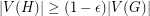.

Keywords: regular; subgraph

## Degenerate colorings of planar graphs ★★★

Author(s): Borodin

A graphis-degenerate if every subgraph ofhas a vertex of degree.

Conjecture   Every simple planar graph has a 5-coloring so that for, the union of anycolor classes induces a-degenerate graph.

Keywords: coloring; degenerate; planar

## Partial List Coloring ★★★

Letbe a simple graph, and for every list assignmentlet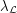be the maximum number of vertices ofwhich are colorable with respect to. Define, where the minimum is taken over all list assignmentswithfor all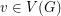.

Conjecture    Letbe a graph with list chromatic numberand. Then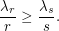Keywords: list assignment; list coloring

## Cube-Simplex conjecture ★★★

Author(s): Kalai

Conjecture   For every positive integer, there exists an integerso that every polytope of dimension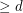has a-dimensional face which is either a simplex or is combinatorially isomorphic to a-dimensional cube.

Keywords: cube; facet; polytope; simplex

## S(S(f)) = S(f) for reloids ★★

Author(s): Porton

Questionfor every endo-reloid?

Keywords: reloid